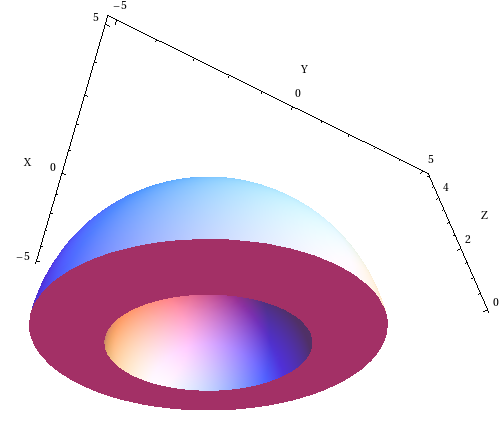# Math Insight

### Image: Half spherical shell domeThe dome region consists of the upper half of a spherical shell with radius from 3 to 5, i.e.,the region is given by \begin{gather*} 9 \le x^2 + y^2 + z^2 \le 25\\ z \ge 0. \end{gather*}

Image file: dome_half_spherical_shell.png

Source image file: dome_half_spherical_shell.nb
Source image type: Mathematica